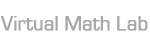Virtual Math Lab is a set of applications designed to support teaching and learning of exact sciences. It originated as a tool for increasing learning efficiency and for making it more attractive, helping students to understand mathematical ideas and their applications.
All applications and visualizations can be used either during traditional blackboard-and-chalk classes to present educational contents or as an interactive element of a blended-learning/e-learning course or an internet website. They serve as a didactic support for both students and teachers at various levels of education.

### Finding the inverse function

This visualization shows how to draw graphs of functions inverse to trigonometric functions y=sin(x), y=cos(x), y=tan(x), y=cot(x) and to the logarithmic function y=ln(x). Additionally, two “trick” methods are shown to help remember the graph of y=arctan(x).

### 2D-Plotter

This application allows to draw graphs of a single variable function. Up to six different graphs can be drawn at a time, each one with a different color.

### 3D-Plotter

This application allows to draw graphs of certain 2-variable functions. Up to two different surfaces can be drawn at a time, each one with a different color. Parameters within the formulas can be easily changed with sliders placed below the function choice box. In order to see a curve resulting from the intersection of two surfaces, one must choose the grid optionfor at least one surface.

### Composite function derivative

This application helps calculate the first derivative of a composite function. The function formula can be entered in the top left corner. On the right-hand-side, one can see the consecutive stages of calculating the first derivative.

### Geometric interpretation of a derivative

This visualization shows the geometric interpretation of the first derivative of a single variable function – several examples are available. The function choice box is placed in the top left corner. Information concerning the range of the slope of a line tangent to the function graph is also provided.

### Asymptotes

This presentation shows several examples of determining, whether a single function variable has any asymptotes. Based on a chosen example, the student can go through several stages of looking for the correct solution.

### Approximating functions with Taylor’s polynomial

This application helps approximate several functions with Taylor’s polynomial. As the degree of the polynomial increases, one can observe as the approximation becomes more precise and the chosen function is reconstructed over a wider domain. The function choice box is located in the upper left corner. The number of terms in a polynomial can be adjusted with the help of a slider in the upper part of the screen. Additionally, after clicking on the calculator iconin the top right corner, one can calculate an exact value and an approximation of a function for a given value of x.

### Geometric applications of an integral

The purpose of this application is to acquaint the user with applications of a definite integral using numerical integration. Three topics are available: “Area”, “Volume” and “Length of a curve”. Within each of the tabs several examples are provided to show consecutive stages of approximating a chosen integral. Additionally, an exact result and approximated value are given together with an absolute and a relative error calculated with a method appropriate for a chosen topic.

### Analytic geometry

This set of visualization shows various methods of solving problems in the field of analytic geometry in a three-dimensional space. Each visualization can be used to illustrate a solution to any of the following exercises on determining:

• a plane running through three points
• a line resulting from an intersection of two planes
• the distance between two parallel lines (two methods are given)
• the distance between two skew lines (two methods are given)
• a point symmetrical with respect to a line
• a point symmetrical with respect to a plane